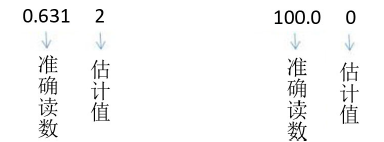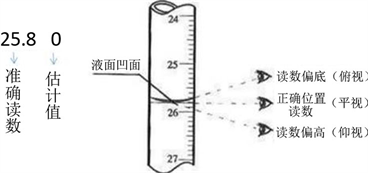﻿ 分析化学实验数据处理教学方法

分析化学实验数据处理教学方法The Teaching Method of Data Processing in Analytical Chemistry Experiment

Abstract: The data processing in the analytical chemistry experiment includes the detailed observation of the experimental phenomena, the accurate record of the experimental data and the analysis and calculation of the test data. Students’ mastery of data processing can not only improve students’ mastery of the basic knowledge of chemistry, but also reflect their ability of generalizing and summarizing the analysis problems. This paper discusses and explores the teaching of data pro-cessing, and expects students to own a solid foundation for future courses and practical work in the future.

1. 引言

2. 数据记录表格的绘制

${m}_{{H}_{2}{C}_{2}{O}_{4}\cdot 2{H}_{2}O}$ ： g， ${c}_{{H}_{2}{C}_{2}{O}_{4}\cdot 2{H}_{2}O}$ ： mol·L−1

3. 分析实验教学中数据的准确记录Table 1. The calibration of sodium hydroxide solution concentrationTable 2. The determination of hydrochloric acid solution concentration

4. 结果的计算

${c}_{{H}_{2}{C}_{2}{O}_{4}\cdot 2{H}_{2}O}=\frac{{m}_{\left({H}_{2}{C}_{2}{O}_{4}\cdot 2{H}_{2}O\right)}×1000\text{\hspace{0.17em}}\text{mL}\cdot {\text{L}}^{-1}}{{M}_{\left({H}_{2}{C}_{2}{O}_{4}\cdot 2{H}_{2}O\right)}×{V}_{\left({H}_{2}{C}_{2}{O}_{4}\cdot 2{H}_{2}O\right)}}$ (1)

${c}_{\left(NaOH\right)}=\frac{{c}_{\left({H}_{2}{C}_{2}{O}_{4}\cdot 2{H}_{2}O\right)}{V}_{\left({H}_{2}{C}_{2}{O}_{4}\cdot 2{H}_{2}O\right)}×2}{{V}_{\left(NaOH\right)}}$ (2)Figure 1. The diagram of data recordingFigure 2. The diagram of burette scale data recording

${c}_{\left(HCl\right)}=\frac{{c}_{\left(NaOH\right)}{V}_{\left(NaOH\right)}}{{V}_{\left(HCl\right)}}$ (3)

${d}_{i}={c}_{\left(NaOH\right)}-{\stackrel{¯}{c}}_{\left(NaOH\right)}$${d}_{i}={c}_{\left(HCl\right)}-{\stackrel{¯}{c}}_{\left(HCl\right)}$ (4)

${d}_{r}=\frac{{d}_{i}}{\stackrel{¯}{c}}×100%$ (5)

5. 结语

 崔学桂, 张晓丽, 胡清萍. 基础化学实验(I)—无机及分析化学实验[J]. 北京: 化学工业出版社, 2015.

 王英华, 魏士刚, 徐家宁. 基础化学实验(化学分析实验分册) [M]. 北京: 高等教育出版社, 2015.

 武汉大学. 分析化学(上册) [M]. 第6版. 北京: 高等教育出版社, 2016.

Top